# RD Sharma Class 10 Ex 10.2 Solutions Chapter 10 Circles

In this chapter, we provide RD Sharma Class 10 Ex 10.2 Solutions Chapter 10 Circles for English medium students, Which will very helpful for every student in their exams. Students can download the latest RD Sharma Class 10 Ex 10.2 Solutions Chapter 10 Circles pdf, Now you will get step by step solution to each question.

# Chapter 10: Circles Exercise – 10.2

### Question: 1

If PT is a tangent at T to a circle whose center is 0 and OP = 17 cm, OT = 8 cm. Find the length of tangent segment PT.

### Solution:

OT = radius = 8 cm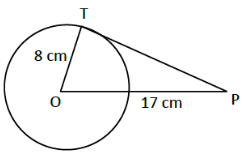OP = 17 cm

PT = length of tangent =?

T is point of contact. We know that at point of contact tangent and radius are perpendicular.

∴ OTP is right angled triangle ∠OTP = 90°, from Pythagoras theorem OT2 + PT2 = OP2

82 + PT= 172

∴ PT = length of tangent = 15 cm.

### Question: 2

Find the length of a tangent drawn to a circle with radius 5cm, from a point 13 cm from the center of the circle.

### Solution:

Consider a circle with center O.

OP = radius = 5 cm.

A tangent is drawn at point P, such that line through O intersects it at Q, OB = 13cm.

Length of tangent PQ =?A + P, we know that tangent and radius are perpendicular.

∆OPQ is right angled triangle, ∠OPQ = 90°

By Pythagoras theorem, OQ2 = OP2 + PQ2

⇒ 132 = 52 + PQ2

⇒ PQ2 = 169 – 25 = 144

⇒ PQ = √114

= 12 cm

Length of tangent = 12 cm

### Question: 3

A point P is 26 cm away from 0 of circle and the length PT of the tangent drawn from P to the circle is 10 cm. Find the radius of the circle.

### Solution:

Given OP = 26 cm

PT = length of tangent = 10 cm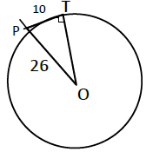At point of contact, radius and tangent are perpendicular ∠OTP = 90°, ∆OTP is right angled triangle. By Pythagoras theorem,

OP2 = OT2 + PT2

262 = OT2 + 102

= 24 cm

OT = length of tangent = 24 cm

### Question: 4

If from any point on the common chord of two intersecting circles, tangents be drawn to circles, prove that they are equal.

### Solution:

Let the two circles intersect at points X and Y.

XY is the common chord.

Suppose ‘A’ is a point on the common chord and AM and AN be the tangents drawn A to the circle

We need to show that AM = AN.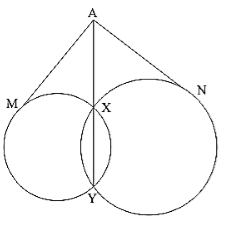In order to prove the above relation, following property will be used.

“Let PT be a. tangent to the circle from an external point P and a secant to the circle through P intersects the circle at points A and B, then PT2 = PA × PB”

Now AM is the tangent and AXY is a secant ∴ AM2 = AX × AY … (i)

AN is a tangent and AXY is a secant

∴ AN2 = AX × AY …. (ii)

From (i) & (ii), we have AM2 = AN2

∴ AM = AN

### Question: 5

If the quadrilateral sides touch the circle prove that sum of pair of opposite sides is equal to the sum of other pair.

### Solution:

Consider a quadrilateral ABCD touching circle with center O at points E, F, G and 11 as in figure.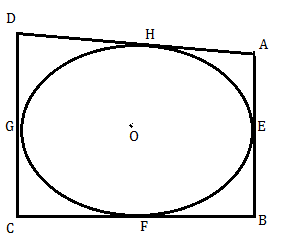We know that

The tangents drawn from same external points to the circle are equal in length.

1. Consider tangents from point A [AM ⊥ AE]

AH = AE … (i)

2. From point B [EB & BF]

BF = EB … (ii)

3. From point C [CF & GC]

FC = CG … (iii)

4. From point D [DG & DH]

DH = DG …. (iv)

Adding (i), (ii), (iii), & (iv)

(AH + BF + FC + DH) = [(AC + CB) + (CG + DG)]

⟹ (AH + DH) + (BF + FC) = (AE + EB) + (CG + DG)

⟹ AD + BC = AB + DC [from fig.]

Sum of one pair of opposite sides is equal to other.

### Question: 6

If AB, AC, PQ are tangents in Fig. and AB = 5 cm find the perimeter of ∆APQ.

### Solution:

Perimeter of AAPQ, (P) = AP + AQ + PQ

= AP + AQ + (PX + QX)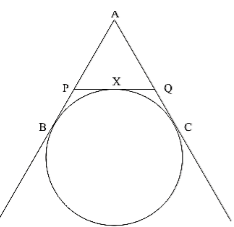We know that

The two tangents drawn from external point to the circle are equal in length from point A,

AB = AC = 5 cm

From point P, PX = PB

From point Q, QX = QC

Perimeter (P) = AP + AQ + (PB + QC)

= (AP + PB) + (AQ + QC)

= AB + AC = 5 + 5

= 10 cm.

### Question: 7

Prove that the intercept of a tangent between two parallel tangents to a circle subtends a right angle at center.

### Solution:

Consider circle with center ‘O’ and has two parallel tangents through A & B at ends of diameter.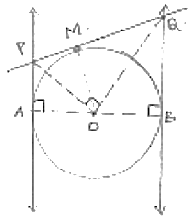Let tangents through M intersects the tangents parallel at P and Q required to prove is that LPOQ = 90°. From fig. it is clear that ABQP is a quadrilateral

∠A + ∠B = 90° + 90° = 180° [At point of contact tangent & radius are perpendicular]

∠A + ∠B + ∠P + ∠Q = 360° [Angle sum property]

∠P + ∠Q = 360° – 180° = 180° … (i)

At P & Q ∠APO = ∠OPQ =1/2 ∠P

∠BQO = ∠PQO = 1/2 ∠Q    in (i)

2∠OPQ + 2∠PQO = 180°

∠OPQ + ∠PQO = 90° …  (ii)

In ∆OPQ, ∠OPQ + ∠PQO + ∠POQ = 180° [Angle sum property]

90° + ∠POQ = 180°           [from (ii)]

∠POQ = 180° – 90° = 90°

∠POQ = 90°

### Question: 8

In Fig below, PQ is tangent at point R of the circle with center O. If ∠TRQ = 30°. Find LPRS.

### Solution:

Given ∠TRQ = 30°.

At point R, OR ⊥ RQ.

∠ORQ = 90°

⟹ ∠TRQ + LORT = 90°

⟹ ∠ORT = 90°- 30° = 60°

ST is diameter, LSRT = 90° [ ∵ Angle in semicircle = 90°]

∠ORT + ∠SRO = 90°

∠SRO + ∠PRS = 90°

∠PRS = 90°- 30° = 60°

### Question: 9

If PA and PB are tangents from an outside point P. such that PA = 10 cm and LAPB = 60°. Find the length of chord AB.

### Solution:

AP = 10 cm ∠APB = 60°

Represented in the figureWe know that

A line drawn from center to point from where external tangents are drawn divides or bisects the angle made by tangents at that point ∠APO = ∠OPB =1/2 × 60° = 30°

The chord AB will be bisected perpendicularly

∴ AB = 2AM

In ∆AMP,

AM = AP sin 30°

AP/2 = 10/2 = 5cm

AP = 2 AM =10 cm … Method (i)

In ∆AMP, ∠AMP = 90°, ∠APM = 30°

∠AMP + ∠APM + ∠MAP = 180°

90° + 30° + ∠MAP = 180°

∠MAP = 180°

In ∆PAB, ∠MAP = ∠BAP = 60°, ∠APB = 60°

We also get, ∠PBA = 60°

∴ ∆PAB is equilateral triangle

AB = AP = 10 cm … Method (ii)

All Chapter RD Sharma Solutions For Class10 Maths

I think you got complete solutions for this chapter. If You have any queries regarding this chapter, please comment on the below section our subject teacher will answer you. We tried our best to give complete solutions so you got good